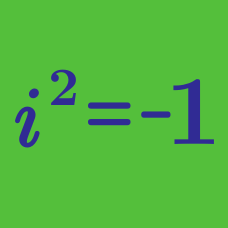Algebra

# Complex Conjugates - Arithmetic

The expression $\displaystyle \frac{1}{2-11i}$ can be rewritten with a real denominator (using the complex conjugate) in the form $\displaystyle \frac{a+bi}{c},$

where $a$, $b$, and $c$ are positive, co-prime integers. What is the sum of $a$, $b$, and $c$?

Details and assumptions

$i$ is the the imaginary unit, defined by $i^2 = -1$.

Consider the complex numbers $A=12+i \mbox{ and } B=11-3i.$ If the value of $2A\overline{A}+2\overline{A}B+A\overline{B}+B\overline{B}$ is $X+Yi$, what is the value of $X-Y$?

Details and assumptions

$\overline{A}$ denotes the complex conjugate of the complex number $A$.

$i$ is the imaginary number that satisfies $i^2 = -1$.

For complex numbers $\alpha=5+7i$ and $\beta=3-i,$ what is the value of $\alpha \overline{\alpha} +\overline{\alpha} \beta +\alpha \overline{\beta} +\beta \overline{\beta}?$

Details and assumptions

$\overline{\alpha}$ denotes the complex conjugate of the complex number $\alpha$.

$i$ is the imaginary number that satisfies $i^2 = -1$.

Consider the complex numbers $A=8-2i \mbox{ and } B=4-i.$ What is the value of $(A+B)^2+\left(\overline{A+B}\right)^2$?

Details and assumptions

$\overline{A}$ denotes the complex conjugate of the complex number $A$.

$i$ is the imaginary number that satisfies $i^2 = -1$.

Let $\omega$ and $z$ be the complex numbers $\omega =2-i, \quad z =\frac{\omega+4}{4\omega-1}.$

If $z\overline{z}$ can be expressed as $\frac{a}{b},$ where $a$ and $b$ are coprime positive integers, what is $a+b?$

Details and assumptions

$\overline{z}$ denotes the complex conjugate of the complex number $z$.

$i$ is the imaginary number that satisfies $i^2 = -1$.

×